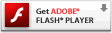# Interactive Generative Art Series – 03 – Gradient ColorsWith the full range of colors randomly available to me, I wanted to get a more fluid randomly created color. I see the hard line breaking one color from the next in the generative art 02 random color experiment. I wanted to have the lines drawn between my 2 points to be a gradient of two colors rather than solid color followed by solid color. In this attempt at a smoother color transition, I needed to better understand the gradientBoxMatrix and createGradientBox. I knew visually what I wanted to accomplish but had to brush up on the docs and then experiment a bit to get the math right. The hard line color change still shows in places, but it’s only when one line is short enough and then is overwritten by the beginning of the next line, so technically it’s doing what I intended, but visually it’s still not as gradual as I was wanting. I could toy a bit more with the rate of color change, but I feel pretty accomplished after having figured out that trigonometry and arc-tangent.

### 03 Gradient Color, play here

Please visit the blog article to view this interactive flash content. Flash plug-in required:### actionscript source code

[cc lang=”actionscript”]
var ball:Sprite = new Sprite();

ball.graphics.beginFill(0x333333, 1);
ball.graphics.drawCircle(0, 0, 30);
ball.graphics.endFill();

var oldx:Number = ball.x;
var oldy:Number = ball.y;

var div:Number = .1;

var ax:Number = 0;
var ay:Number = 0;

var line_max_width:Number = 75;
var line_min_width:Number = 5;
var line_width:Number = randomRange(line_min_width, line_max_width);
var line_width_velocity:Number = 0;
var dampen:Number = 0.95;

var colors:Object = new Object();
colors.r = 0;
colors.g = 122;
colors.b = 255;
colors.rv = 0;
colors.gv = 0;
colors.bv = 0;
colors.min = 0;
colors.max = 155;
var color_first:Number = 0x000000;
var color_second:Number = rgb2hex(colors.r, colors.g, colors.b);

function loop () {
oldx = ball.x;
oldy = ball.y;

ball.x -= ax = (ax + (ball.x – mouseX) * div) * .9;
ball.y -= ay = (ay + (ball.y – mouseY) * div) * .9;

line_width_velocity += randomRangeAxis(3);
line_width += line_width_velocity;
line_width_velocity *= dampen;
if(line_width > line_max_width) { line_width = line_max_width; }
if (line_width < line_min_width) { line_width = line_min_width; }

color_first = color_second;
color_step();
color_second = rgb2hex(colors.r, colors.g, colors.b);

var dx:Number = ball.x – oldx;
var dy:Number = ball.y – oldy;
this.graphics.lineStyle(line_width);
this.graphics.lineTo(ball.x, ball.y);
}

setInterval(loop, 1000/30);

function rgb2hex(r:Number, g:Number, b:Number):Number {
return(r<<16 | g<<8 | b); } function color_step(){ colors.rv += randomRangeAxis(10); colors.r += colors.rv; colors.rv *= dampen; if (colors.r > colors.max) {
colors.r = colors.max;
} else if (colors.r < colors.min){ colors.r = colors.min; } colors.gv += randomRangeAxis(10); colors.g += colors.gv; colors.gv *= dampen; if (colors.g > colors.max) {
colors.g = colors.max;
} else if (colors.g < colors.min){ colors.g = colors.min; } colors.bv += randomRangeAxis(10); colors.b += colors.bv; colors.bv *= dampen; if (colors.b > colors.max) {
colors.b = colors.max;
} else if (colors.b < colors.min){
colors.b = colors.min;
}
}
//random number between min and max
function randomRange(max:Number, min:Number = 0):Number {
return Math.random() * (max – min) + min;
}
//random number range centered at 0 with the specified max, randomRange(-max, max)
function randomRangeAxis(max:Number):Number {
return Math.random() * (max * 2) – max;
}
[/cc]
You’ll see I introduced a few new methods into this example. I wanted to simplify my random number generation so I created a couple separate functions to create a random number within a certain range, one given a min and max and another to give a random range around an axis, so for example a number between -10 and +10.